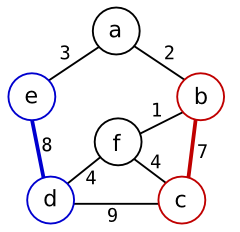# Maximum Weighted Matching

Good algorithms for maximum weighted matching in general graphs have been known for decades. Although free software implementations of these algorithms are available on the web, they are not so easy to find. This web page presents a new implementation of maximum weighted matching in Python and in Perl. It also links to a number of existing library implementations.

### Matching in a graph

In graph theory, a matching is a subset of edges such that none of the selected edges share a common vertex .

A maximum cardinality matching is a matching that contains the largest possible number of edges (or equivalently, the largest possible number of vertices). A perfect matching is a matching which covers all vertices.With respect to a weighted graph, a maximum weight matching is a matching for which the sum of the weights of the matched edges is as large as possible.

In the depicted graph, a matching of weight 15 can be found by pairing vertex b to vertex c and vertex d to vertex e (leaving vertices a and f unpaired).

Matching algorithms have applications in operations research. In my case, the problem is to schedule rounds of games in a chess-style tournament. For each round, I need to find pairs of participants such that no pair has already played against each other in a previous round. Within those constraints, I want to maximize some measure of usefulness (for example, choose opponents of similar strength).

### Algorithms

Finding a maximum weight matching is far from trivial. A few complications are already apparent from the graph in the figure. The maximum weight matching in this graph covers only 4 out of 6 vertices; even though there exist perfect matchings for this graph, none of those achieves the maximum weight. Note also that the single edge with maximum weight (the edge between c and d with weight 9) is not part of the maximum weight matching.

The first polynomial time algorithm for maximum matching was found by Edmonds  and subsequently improved by Gabow and others . Currently known algorithms can find maximum weighted matchings for dense graphs in time O(n3). For sparse graphs, there are faster algorithms that run within O(nm logn) .

Simpler and faster algorithms can be applied if the problem is restricted to bipartite graphs. Consequently, many graph libraries provide separate solvers for matching in bipartite graphs. Implementations of bipartite matching are also easier to find on the web than implementations for general graphs.

### My implementation

Since I did not find any Perl implementations of maximum weighted matching, I lightly decided to write some code myself. It turned out that I had underestimated the problem, but by the time I realized my mistake, I was so obsessed with the problem that I refused to give up.

My code implements an O(n3) algorithm described by Galil . It computes a maximum weight matching in a general graph (not necessarily bipartite). Alternatively, it can compute a maximum cardinality matching with largest weight among all maximum cardinality matchings.

Description File
Standalone Python code. Prototype for the other versions.
(Updated 2013-04-07 to support Python 3,
thanks to Daniel Saunders.)
mwmatching.py
Python 2 code, refactored to fit in the NetworkX library.
(Updated 2009-07-31 to work with NetworkX v0.99,
thanks to Leonid Chindelevitsch.)
matching.py
Doctests for the NetworkX variant of the Python code. matching.txt
Perl 5 module Graph::Matching.
(Also available from CPAN.)
Graph-Matching-0.02.tar.gz

NetworkX is a pure Python library for network analysis. This matching algorithm is included in recent versions of NetworkX under `networkx.algorithms.matching`.

### Other (free) implementations

Ed Rothberg implemented Gabow's O(n3) weighted matching algorithm in C. Code is available from the DIMACS archive: ftp://dimacs.rutgers.edu/pub/netflow/. This is mostly a proof-of-concept implementation without a nice application interface.

LEMON is a C++ template library of graph algorithms. It contains an O(nm logn) implementation of Edmond's maximum weighted matching algorithm.

GOBLIN is a C++ class library of graph algorithms. It contains a maximum matching algorithm based on reduction to a maximum balanced flow problem.

The speed of `mwmatching.py` is largely determined by overhead of the Python interpreter. A 10x speedup can be achieved by compiling the algorithm to native code with Shedskin, a Python-to-C++ compiler.

### References

• Wikipedia: Matching.
• Jack Edmonds, Paths, trees, and flowers, Canadian Journal of Mathematics, 1965.
• H.J. Gabow, Implementation of algorithms for maximum matching on nonbipartite graphs, Stanford Ph.D thesis, 1973.
• Zvi Galil, Efficient algorithms for finding maximum matching in graphs, ACM Computing Surveys, 1986.
• Snjólfur Ólafsson, Weighted Matching in Chess Tournaments, Journal of the Operational Research Society, 1990.